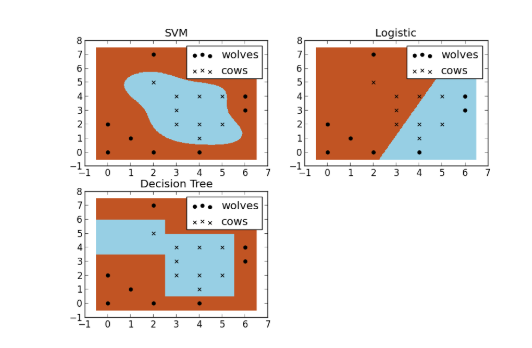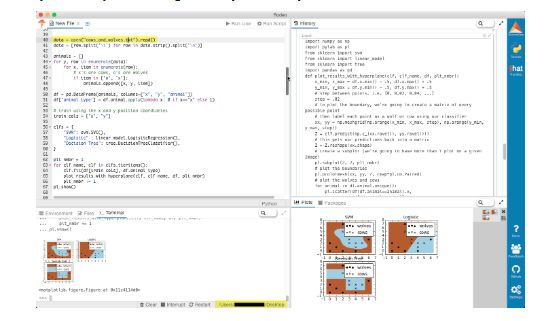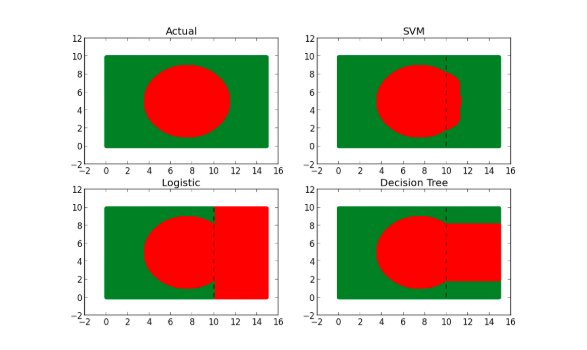>科技>正文

# 【ML】支持向量机是什么？我为什么要使用它?

1 支持向量机是什么？如何理解？1# Data driven farmer goes to the Rodeo

2

3importnumpy asnp

4importpylab aspl

5fromsklearn importsvm

6fromsklearn importlinear_model

7fromsklearn importtree

8importpandas aspd

9

10

11defplot_results_with_hyperplane(clf, clf_name, df, plt_nmbr):

12x_min, x_max = df.x.min() - .5, df.x.max() + .5

13y_min, y_max = df.y.min() - .5, df.y.max() + .5

14

15# step between points. i.e. [0, 0.02, 0.04, ...]

16step = .02

17# to plot the boundary, we're going to create a matrix of every possible point

18# then label each point as a wolf or cow using our classifier

19xx, yy = np.meshgrid(np.arange(x_min, x_max, step), np.arange(y_min, y_max, step))

20Z = clf.predict(np.c_[xx.ravel(), yy.ravel()])

21# this gets our predictions back into a matrix

22Z = Z.reshape(xx.shape)

23

24# create a subplot (we're going to have more than 1 plot on a given image)

25pl.subplot( 2, 2, plt_nmbr)

26# plot the boundaries

27pl.pcolormesh(xx, yy, Z, cmap=pl.cm.Paired)

28

29# plot the wolves and cows

30foranimal indf.animal.unique():

31pl.scatter(df[df.animal==animal].x,

32df[df.animal==animal].y,

33marker=animal,

34label= "cows"ifanimal== "x"else"wolves",

35color= 'black')

36pl.title(clf_name)

37pl.legend(loc= "best")

38

39

40data = open( "cows_and_wolves.txt").read()

41data = [row.split( 't') forrow indata.strip().split( 'n')]

42

43animals = []

44fory, row inenumerate(data):

45forx, item inenumerate(row):

46# x's are cows, o's are wolves

47ifitem in[ 'o', 'x']:

48animals.append([x, y, item])

49

50df = pd.DataFrame(animals, columns=[ "x", "y", "animal"])

51df[ 'animal_type'] = df.animal.apply( lambdax: 0ifx== "x"else1)

52

53# train using the x and y position coordiantes

54train_cols = [ "x", "y"]

55

56clfs = {

57"SVM": svm.SVC(),

58"Logistic": linear_model.LogisticRegression(),

59"Decision Tree": tree.DecisionTreeClassifier(),

60}

61

62plt_nmbr = 1

63forclf_name, clf inclfs.iteritems():

64clf.fit(df[train_cols], df.animal_type)

65plot_results_with_hyperplane(clf, clf_name, df, plt_nmbr)

66plt_nmbr += 1

67pl.show()

• 逻辑模型
• 决策树
• SVM1importnumpy asnp

2importpylab aspl

3importpandas aspd

4

5fromsklearn importsvm

6fromsklearn importlinear_model

7fromsklearn importtree

8

9fromsklearn.metrics importconfusion_matrix

10

11x_min, x_max = 0, 15

12y_min, y_max = 0, 10

13step = .1

14# to plot the boundary, we're going to create a matrix of every possible point

15# then label each point as a wolf or cow using our classifier

16xx, yy = np.meshgrid(np.arange(x_min, x_max, step), np.arange(y_min, y_max, step))

17

18df = pd.DataFrame(data={ 'x': xx.ravel(), 'y': yy.ravel()})

19

20df[ 'color_gauge'] = (df.x -7.5)** 2+ (df.y -5)** 2

21df[ 'color'] = df.color_gauge.apply( lambdax: "red"ifx <= 15else"green")

22df[ 'color_as_int'] = df.color.apply( lambdax: 0ifx== "red"else1)

23

24print"Points on flag:"

25printdf.groupby( 'color').size()

26print

27

28figure = 1

29

30# plot a figure for the entire dataset

31forcolor indf.color.unique():

32idx = df.color==color

33pl.subplot( 2, 2, figure)

34pl.scatter(df[idx].x, df[idx].y, color=color)

35pl.title( 'Actual')

36

37

38train_idx = df.x < 10

39

40train = df[train_idx]

41test = df[-train_idx]

42

43

44print"Training Set Size: %d"% len(train)

45print"Test Set Size: %d"% len(test)

46

47# train using the x and y position coordiantes

48cols = [ "x", "y"]

49

50clfs = {

51"SVM": svm.SVC(degree= 0.5),

52"Logistic": linear_model.LogisticRegression(),

53"Decision Tree": tree.DecisionTreeClassifier()

54}

55

56

57# racehorse different classifiers and plot the results

58forclf_name, clf inclfs.iteritems():

59figure += 1

60

61# train the classifier

62clf.fit(train[cols], train.color_as_int)

63

64# get the predicted values from the test set

65test[ 'predicted_color_as_int'] = clf.predict(test[cols])

66test[ 'pred_color'] = test.predicted_color_as_int.apply( lambdax: "red"ifx== 0else"green")

67

68# create a new subplot on the plot

69pl.subplot( 2, 2, figure)

70# plot each predicted color

71forcolor intest.pred_color.unique():

72# plot only rows where pred_color is equal to color

73idx = test.pred_color==color

74pl.scatter(test[idx].x, test[idx].y, color=color)

75

76# plot the training set as well

77forcolor intrain.color.unique():

78idx = train.color==color

79pl.scatter(train[idx].x, train[idx].y, color=color)

80

81# add a dotted line to show the boundary between the training and test set

82# (everything to the right of the line is in the test set)

83#this plots a vertical line

84train_line_y = np.linspace(y_min, y_max) #evenly spaced array from 0 to 10

85train_line_x = np.repeat( 10, len(train_line_y)) #repeat 10 (threshold for traininset) n times

86# add a black, dotted line to the subplot

87pl.plot(train_line_x, train_line_y, 'k--', color= "black")

88

89pl.title(clf_name)

90

91print"Confusion Matrix for %s:"% clf_name

92printconfusion_matrix(test.color, test.pred_color)

93pl.show()

• SVM新手教程:麻省理工学院的Zoya Gavrilov教授的一些基础知识
• 初学者SVM算法的工作原理：ThalesSehnKörting的视频
• 来自纽约大学和范德比尔特生物医学的中级支持向量机介绍
https://www.kdnuggets.com/2017/02/yhat-support-vector-machine.html